## College Physics with Mastering Physics

Physics & Astronomy

## Quiz 10 : Temperature and Kinetic TheoryLooking for Introductory Physics Homework Help?# Quiz 10 : Temperature and Kinetic Theory

Heat always flows from a hot body (high temperature) to cold body (low temperature), if they are in thermal contact. When a body is heated its internal energy increases, motion of inner particles of body increases. But internal energy is function of mass and specific heat too. Mass and specific heat depends on composition of material may be different for different materials. Suppose a material has high temperature but the values of its mass and specific heat are less that means it has low internal energy and another material has low temperature more mass and specific heat. If these two are in thermal contact heat will flow from material of high temperature to low temperature. So it is not necessary that heat always flow from a body of more internal energy to low internal energy.

Given that, a person running a fever has a body temperature of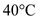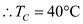By using the relation,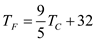We can convert from Celsius to Fahrenheit scale.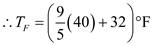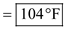The kinetic energy of ideal gases is,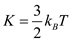Here,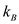is Boltzmann's constant and T is temperature. Root mean square velocity is,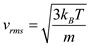In above formula, the kinetic energy is dependent upon the temperature. Also, momentum is,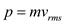Substitute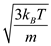for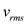,Thus, momentum is also dependent on temperature. Also, potential energy contributes to the total internal energy which is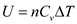Here,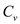is molar heat capacity and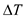denotes the change in temperature. Thus, it also depends upon temperature. Thus, (a), (b) and (c) are incorrect options. Hence, the correct option is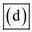.